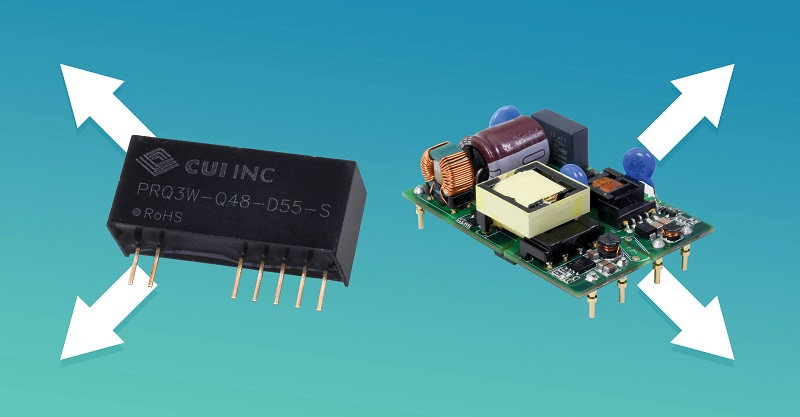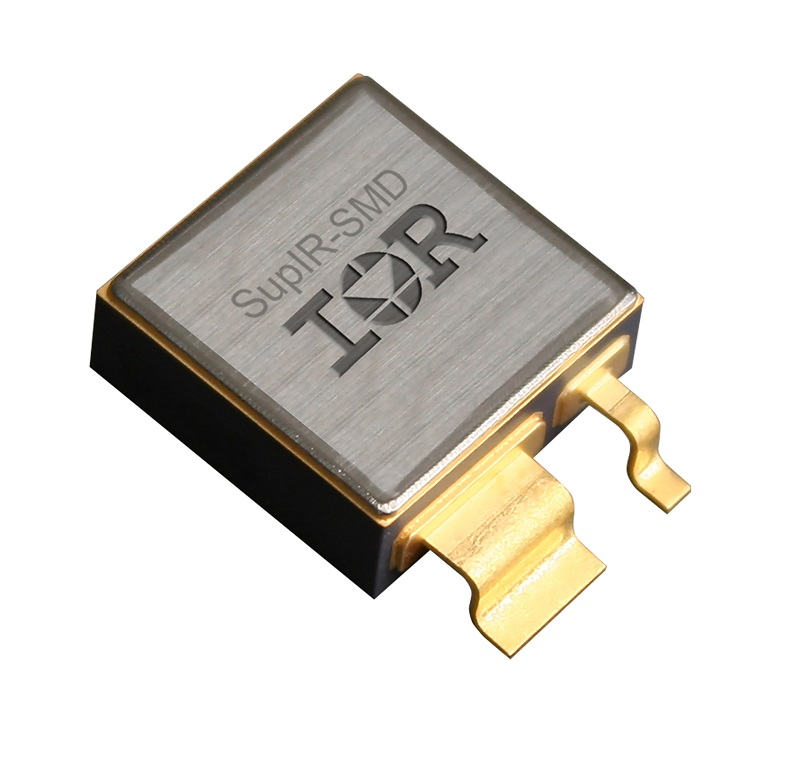# Measuring the power of complex RF waveforms

Author:
Giovanni D’Amore, Keysight Technologies

Date
01/28/2017PDF
porn porntube
##### How different approaches influence measurement outcomes using three examples

The ability to make consistent measurements of RF or microwave power levels is vital to developing effective radio systems and networks. This article explores how different approaches affect the resultant measurements.

The test case compares various approaches to making average power measurements of continuous-wave (CW), multi-tone, 32 QAM (quadrature amplitude modulation), and pulsed signals. A Keysight MXG vector signal generator was used to produce a 6 GHz signal with an output power of -20 dBm.

Power-sensor based measurements used a Keysight U2000A USB power sensor connected to an N9938A FieldFox spectrum analyzer with Option 302. Spectrum-analyzer based measurements used the same FieldFox analyzer, with an integral channel power meter (CPM, Option 310) connected to the signal generator using coaxial cable.

Measuring a CW signal

CW signals do not carry information and so the average power and peak power are the same and the signal has no bandwidth. Measurement equipment can use a narrow filter to improve its sensitivity (noise floor).

Figure 1a shows the swept frequency response of a typical spectrum analyzer, around a tone, across a measurement span of 500 kHz. The signal’s peak (and therefore also average) power is -20.08 dBm. The USB power sensor used in Figure 1b gives -20.00 dBm. The CPM option on the FieldFox, shown in Figure 1c, gives -20.09 dBm.

Click image to enlarge

Figure 1a

Click image to enlarge

Figure 1b

Click image to enlarge

Figure 1c

Figure 1: Measuring a CW signal using (a) spectrum analyzer and marker, (b) power sensor, and (c) spectrum analyzer with CPM (Source: Keysight Technologies)

The three measurements are within 0.1 dB. The power sensor has the highest accuracy, or lowest measurement uncertainty.

Measuring a multi-tone signal

In this example, the power from the signal generator is distributed among five tones at 500 kHz intervals. The peak power of this signal differs from its average power because of the phase relationship between the tones.

If the total power for the tones is -20 dBm (10 μW) then each has a power of 26.98 dBm (2μW). An average power sensor will measure the average power of all five tones, in this case, -20.13 dBm (see Figure 2a-c). The CPM gives a measurement of 20.2 dBm, so long as the CPM’s measurement span is set to include all five tones (in this case, this demands a span of at least 2.5 MHz).

Click image to enlarge

Figure 2a

Clcik image to enlarge

Figure 2b

Click image to enlarge

Figure 2c

Figure 2: Measuring a multi-tone signal using the (a) spectrum analyzer, (b) power sensor, and (c) spectrum analyser with CPM (Source: Keysight Technologies)

Power sensors can’t make measurements of single tones or specific measurement spans, because they are not frequency selective. Peak power measurements usually need a sensor that can handle peak powers and a filter wide enough to capture all the tones at once.

Measuring a digitally modulated signal

The digitally modulated signal shown here uses 32 QAM with a symbol rate of 5 Msymbol/s. The symbols are filtered, so the signal bandwidth is approximately 8 MHz (see Figure 3a). The power sensor reports an average power of -20.00 dBm (Figure 3b), and the CPM reports -20.07 dBm (Figure 3c).

Click image to enlarge

Figure 3a

Click image to enlarge

Figure 3b

Click image to enlarge

Figure 3c

Figure 3: Measuring a 32 QAM signal using the (a) spectrum analyzer, (b) power sensor, and (c) tuned receiver with CPM (Source: Keysight Technologies)

A power sensor will report the correct average power of a signal with unknown bandwidth, so long as its power and frequency are within the sensor’s capabilities. To make accurate measurements with the FieldFox you need to set the correct measurement span on its CPM. This can be done by using a standard spectrum analyzer to discover the signal’s bandwidth, and then switching in the CPM once it has the correct span setting.

Measuring pulsed waveforms

In pulsed waveforms, the ratio of the pulse width (how long the pulse is on) to the time between the pulses (its period) is its duty factor (DF). The peak power of such waveforms is the average power divided by the DF, given a repeating pulse-train. Figure 4 shows the measured spectrum and average power of a pulsed waveform with a 20 μs pulse width and a 20% DF.

Figure 4a shows the spectrum analyzer display of a typical “sin(x)/x” response as a function of frequency. Sidebands in the frequency domain continue outside the displayed 1 MHz frequency span. The average power using the power sensor (Figure 4b) is reported as -27.01 dBm. The CPM (Figure 4c) reports an average power of -26.8 dBm.

Click image to enlarge

Figure 4a

Click image to enlarge

Figure 4b

Click image to enlarge

Figure 4c

Figure 4: Measuring a pulsed waveform with 20% duty factor using the (a) spectrum analyzer, (b) power sensor, and (c) spectrum analyzer with CPM (Source: Keysight Technologies)

For the CPM measurement, the span was set to 3 MHz to capture most of the waveform’s sideband energy (increasing the span beyond 3 MHz did not change the measured power.) The peak power for this waveform with a 20% DF can be calculated by increasing the average level by 6.99 dB (10log(1/DF)). Using a measured average power of -27 dBm, the peak power is calculated as -20 dBm — which is what the signal generator was set to produce.

Detection and filtering in peak power measurements

The block diagram for a peak power sensor/meter is like that of an average power sensor (Figure 5), although the peak power sensor/meter has a wider bandwidth and higher sample rates in its ADC to capture rapid transitions in pulsed and complex modulated waveforms.

Click image to enlarge

Figure 5: Block diagram of a peak power sensor/meter (Source: Keysight Technologies)

A typical peak power sensor has two measurement paths, optimised for measuring either the average or the peak power. In the peak-power measurement path, the sensor has a two-layer detection scheme.

The first detection occurs when the wideband diode rectifies the incoming waveform, while preserving the shape of its envelope. The signal is then amplified and filtered. The filter’s bandwidth is usually selectable, at up to 30 MHz for the Keysight P-Series and X-Series sensors, and must be greater than the signal under measurement.

The second detection happens after filtering, when the ADC samples the signal (at up to 1 Gsample/s in peak-power meters such as the Keysight 8990). This high sampling rate captures the true shape of the waveform, from which the peak power, pulsewidth, period, rise- and fall time characteristics can be measured or derived.

Making measurements with a peak power sensor

Peak-power sensors can interface with spectrum analyzers to make field measurements of the time domain profile of a pulsed waveform. Figure 6 shows the measured power envelope of a pulsed waveform as a function of time.

Click image to enlarge

Figure 6: Measuring a pulsed waveform using a peak power sensor connected to a spectrum analyzer (Source: Keysight Technologies)

The input waveform is a pulse-modulated 40 GHz signal with a 1 μs pulse width and 10% DF. It was captured using a Keysight N9344C handheld spectrum analyser connected to a U2020 X-Series USB peak and average power sensor.

Using this peak-power sensor, an analyzer can display peak power, average power, and waveform characteristics such as rise and fall times. Markers on the display can be set to show specific timing and power levels in the waveform. The Keysight peak power meters have bandwidths of up to 30 MHz, making it possible to measure waveforms’ rise and fall times down to 13 ns.

This test case has shown that modern power sensors, power meters and spectrum analyzers can make sophisticated measurements on complex signals, but must be deployed with an understanding of their advantages and constraints in order for those measurements to be valid.

### RELATED#### 650V devices added to super junction N-Channel MOSFET series

Aug 5,2020#### Asymmetrical Output, Isolated Dc-Dc Converters

Aug 5,2020#### Multilayer Ceramic Capacitors work up to 150°C

Aug 5,2020Aug 5,2020